@huanghaian 2020-11-18T05:39:22.000000Z 字数 9395 阅读 1258

# mmdetection：RPN分析

mmdetection

## 0 摘要

RPN全称是Region Proposal Network区域提取网络，是Ren等人在2015年提出的一个模型，用以替代Fast RCNN 等两阶段方法中的外部region proposal算法，提高整个检测pipeline的速度。RPN和FasterRCNN是在同一篇 论文中提出的，且RPN是Faster RCNN的一个模块。 但RPN也可以单独使用，在图像上产生物体的 proposal，或者直接用于特定物体的检测。 RPN算法对于理解SSD、YOLO和RetinaNet等单阶段检测方法也有所帮助。通过对比分析可以发现RPN和SSD、RetinaNet非常类似。为了方便理解，本教程也将单独对RPN讲解。

## 1 算法分析

### 1.1 Anchor

Anchor概念是在faster rcnn中第一次提到，是目前anchor-base算法中非常重要的一个超参，其参数设置对最终结果有巨大影响，理解Anchor的核心思想才能完整把握整个算法流程。

Anchor字面意思是锚，是用于固定船的部件，锚点放置在哪个位置，那么船就只能在其约束的范围内飘动。在计算机视觉中也是同一个意思，假设在图片的某个位置设置了一个锚点，该锚点不仅有位置信息还有宽高属性，那么相应的物体bbox预测就受到该锚点的约束(即正负样本属性定义)，相当于提供了先验。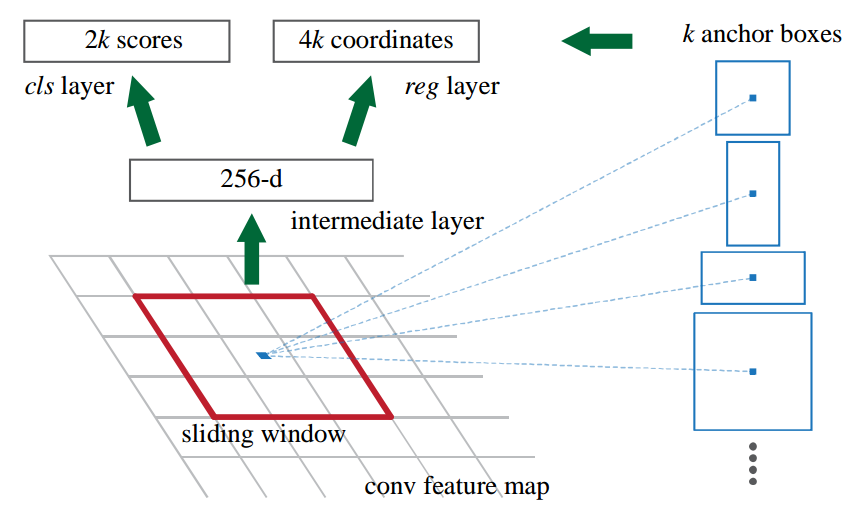### 1.2 backbone

backbone=dict(    type='ResNet',    depth=50,    num_stages=3,    strides=(1, 2, 2),    dilations=(1, 1, 1),    out_indices=(2, ),    frozen_stages=1,    norm_cfg=dict(type='BN', requires_grad=False),    norm_eval=True,    style='caffe'),

self.rpn_conv = nn.Conv2d(1024, 1024, 3, padding=1)self.rpn_cls = nn.Conv2d(1024,self.num_anchors * 1, 1)self.rpn_reg = nn.Conv2d(1024, self.num_anchors * 4, 1)

# 通道变换x = self.rpn_conv(x)x = F.relu(x, inplace=True)# 输出Headrpn_cls_score = self.rpn_cls(x)rpn_bbox_pred = self.rpn_reg(x)return rpn_cls_score, rpn_bbox_pred

### 1.4 正负样本定义

anchor_generator=dict(    type='AnchorGenerator',    scales=[2, 4, 8, 16, 32],    ratios=[0.5, 1.0, 2.0],    strides=),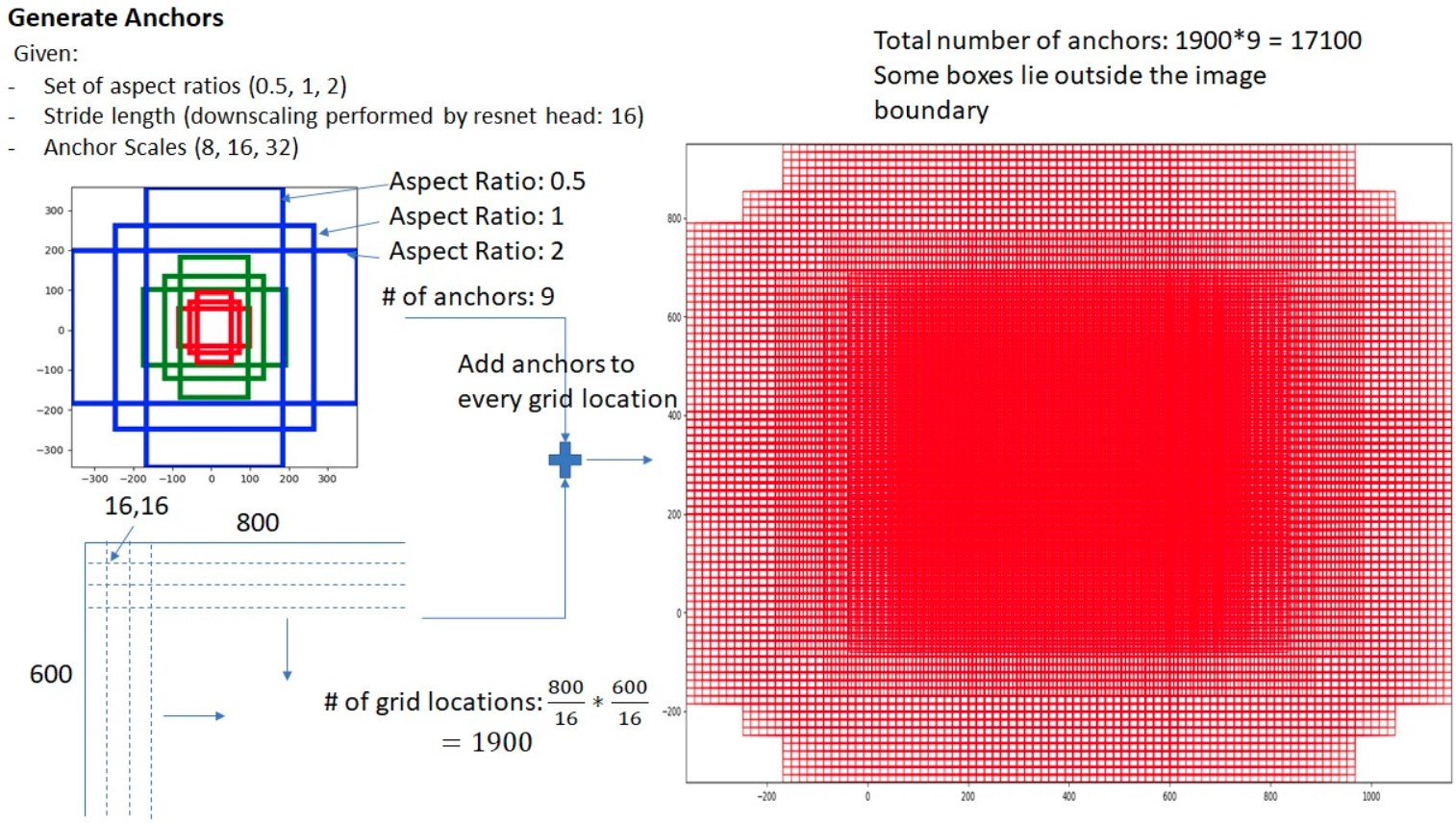AnchorGenerator代码实现分析如下所示：
(1) 先对单个位置(0,0)生成base anchors

# base_size=strides=16w = base_sizeh = base_size# 计算高宽比例h_ratios = torch.sqrt(ratios)w_ratios = 1 / h_ratios# base_size乘上宽高比例乘上尺度，就可以得到5x3=15个anchor的原图尺度wh值ws = (w * w_ratios[:, None] * scales[None, :]).view(-1)hs = (h * h_ratios[:, None] * scales[None, :]).view(-1)# 得到x1y1x2y2格式的base_anchor坐标值base_anchors = [    x_center - 0.5 * ws, y_center - 0.5 * hs, x_center + 0.5 * ws,    y_center + 0.5 * hs]# 堆叠起来即可base_anchors = torch.stack(base_anchors, dim=-1)

(2) 利用输入的特征图大小加上base anchors，得到每个特征图位置的对于原图的anchors

# 特征图大小，这里是(h//16,w//16)feat_h, feat_w = featmap_size# 遍历特征图上所有位置，并且乘上stride，从而变成原图坐标shift_x = torch.arange(0, feat_w, device=device) * strideshift_y = torch.arange(0, feat_h, device=device) * strideshift_xx, shift_yy = self._meshgrid(shift_x, shift_y)shifts = torch.stack([shift_xx, shift_yy, shift_xx, shift_yy], dim=-1)shifts = shifts.type_as(base_anchors)# (0,0)位置的base_anchor，假设原图上坐标shifts，即可得到特征图上面每个点映射到原图坐标上的anchorall_anchors = base_anchors[None, :, :] + shifts[:, None, :]all_anchors = all_anchors.view(-1, 4)return all_anchors

assigner=dict(    type='MaxIoUAssigner',    pos_iou_thr=0.7,    neg_iou_thr=0.3,    min_pos_iou=0.3,    ignore_iof_thr=-1),

MaxIoUAssigner的操作包括4个步骤：

3. 将每个anchor和所有gt bbox计算iou，找出最大iou，如果其最大iou大于等于pos_iou_thr，则设置该anchor的mask设置为1，表示该anchor负责预测该gt bbox，且是高质量anchor
4. 3的设置可能会出现某些gt bbox没有分配到对应的anchor(由于iou低于pos_iou_thr)，故下一步对于每个gt bbox还需要找出和最大iou的anchor位置，如果其iou大于min_pos_iou，将该anchor的mask设置为1，表示该anchor负责预测对应的gt。通过本步骤，可以最大程度保证每个gt bbox都有相应的anchor负责预测，如果其最大iou值还是小于min_pos_iou，那就没办法了，只能当做忽略样本了。从这一步可以看出，3和4有部分anchor重复分配了，即当某个gt bbox和anchor的最大iou大于等于pos_iou_thr，那肯定大于min_pos_iou，此时3和4步骤分配的同一个anchor。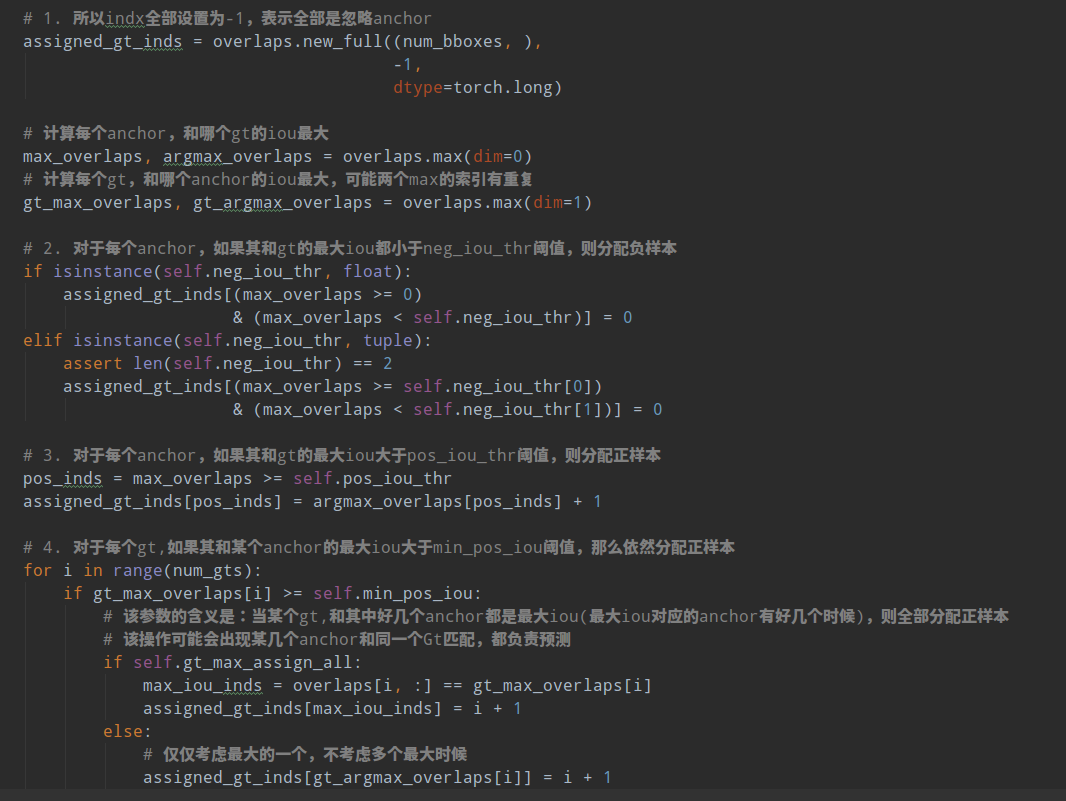### 1.5 正负样本采样

sampler=dict(    type='RandomSampler',    num=256,    pos_fraction=0.5,    neg_pos_ub=-1,    add_gt_as_proposals=False)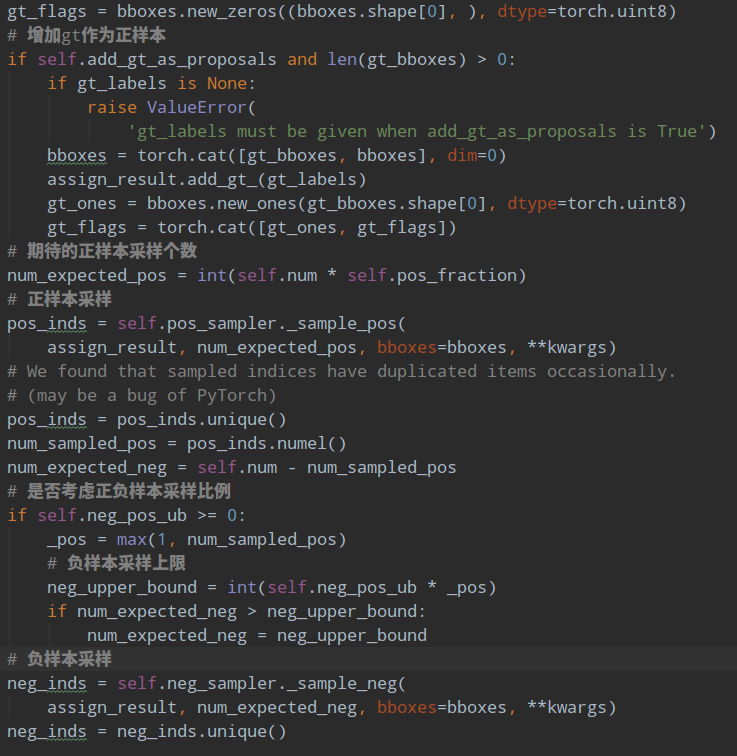# 随机采样正样本def _sample_pos(self, assign_result, num_expected, **kwargs):    """Randomly sample some positive samples."""    pos_inds = torch.nonzero(assign_result.gt_inds > 0, as_tuple=False)    if pos_inds.numel() != 0:        pos_inds = pos_inds.squeeze(1)    if pos_inds.numel() <= num_expected:        return pos_inds    else:        return self.random_choice(pos_inds, num_expected)# 随机采样负样本def _sample_neg(self, assign_result, num_expected, **kwargs):    """Randomly sample some negative samples."""    neg_inds = torch.nonzero(assign_result.gt_inds == 0, as_tuple=False)    if neg_inds.numel() != 0:        neg_inds = neg_inds.squeeze(1)    if len(neg_inds) <= num_expected:        return neg_inds    else:        return self.random_choice(neg_inds, num_expected)

### 1.6 bbox编解码

bbox_coder=dict(    type='DeltaXYWHBBoxCoder',    target_means=[.0, .0, .0, .0],    target_stds=[1.0, 1.0, 1.0, 1.0]),

target_means和target_stds相当于对bbox回归的4个txtytwth进行额外变换，目的是更好的平衡4个输出值。先不考虑target_means和target_stds，其编码公式如下：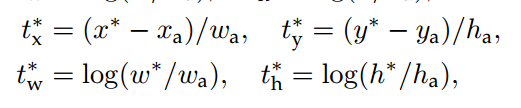$x^*,y^*$是gt bbox的中心xy坐标，$w^*,h^*$是gt bbox的wh值，$x^a,y^a$是anchor的中心xy坐标，$w^a,h^a$是anchor的wh值，$t^*$是bbox分支输出的4个值对应targets，此过程相当于gt bbox编码。容易看出其txty的预测值表示gt bbox中心相对于anchor中心点的偏移，并且通过处于anchor的wh进行归一化；而twth的预测值表示gt bbox的wh除以anchor的wh，然后取log非线性变换即可。

dx = (gx - px) / pwdy = (gy - py) / phdw = torch.log(gw / pw)dh = torch.log(gh / ph)deltas = torch.stack([dx, dy, dw, dh], dim=-1)# 最后减掉均值，处于标准差means = deltas.new_tensor(means).unsqueeze(0)stds = deltas.new_tensor(stds).unsqueeze(0)deltas = deltas.sub_(means).div_(stds)

# 先乘上std，加上meanmeans = deltas.new_tensor(means).view(1, -1).repeat(1, deltas.size(1) // 4)stds = deltas.new_tensor(stds).view(1, -1).repeat(1, deltas.size(1) // 4)denorm_deltas = deltas * stds + meansdx = denorm_deltas[:, 0::4]dy = denorm_deltas[:, 1::4]dw = denorm_deltas[:, 2::4]dh = denorm_deltas[:, 3::4]# wh解码gw = pw * dw.exp()gh = ph * dh.exp()# 中心点xy解码gx = px + pw * dxgy = py + ph * dy# 得到x1y1x2y2的gt 预测坐标x1 = gx - gw * 0.5y1 = gy - gh * 0.5x2 = gx + gw * 0.5y2 = gy + gh * 0.5

### 1.7 loss计算

loss_cls=dict(    type='CrossEntropyLoss', use_sigmoid=True, loss_weight=1.0),loss_bbox=dict(type='L1Loss', loss_weight=1.0)))

## 2 推理流程

rpn=dict(    nms_across_levels=False,    nms_pre=12000,    nms_post=2000,    max_num=2000,    nms_thr=0.7,    min_bbox_size=0))

scores = rpn_cls_score.sigmoid()

2.对rpn_cls_score值进行降序排列，提取前nms_pre个位置预测值，防止后续nms操作由于输入样本过多导致耗时

if cfg.nms_pre > 0 and scores.shape > cfg.nms_pre:    ranked_scores, rank_inds = scores.sort(descending=True)    topk_inds = rank_inds[:cfg.nms_pre]    scores = ranked_scores[:cfg.nms_pre]    rpn_bbox_pred = rpn_bbox_pred[topk_inds, :]    anchors = anchors[topk_inds, :]

3.对保留的位置进行bbox解码，得到原图尺度bbox

proposals = self.bbox_coder.decode(    anchors, rpn_bbox_pred, max_shape=img_shape)

4.对所有解码后bbox进行nms操作，并取分值排名前cfg.nms_post个输出

nms_cfg = dict(type='nms', iou_threshold=cfg.nms_thr)        dets, keep = batched_nms(proposals, scores, ids, nms_cfg)return dets[:cfg.nms_post]

## 3 总结